# Torsion is antisymmetric

(diff) ← Older revision | Latest revision (diff) | Newer revision → (diff)

## Statement

Let$M$ be a differential manifold and$\nabla$ be a linear connection on$M$ (viz.,$\nabla$ is a connection on the tangent bundle$TM$ of$M$).

Consider the torsion of$\nabla$, namely: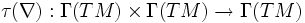$\tau(\nabla): \Gamma(TM) \times \Gamma(TM) \to \Gamma(TM)$

given by: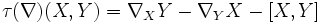$\tau(\nabla)(X,Y) = \nabla_X Y - \nabla_Y X - [X,Y]$

Then,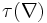$\tau(\nabla)$ is antisymmetric, i.e.: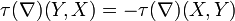$\tau(\nabla)(Y,X) = -\tau(\nabla)(X,Y)$

Equivalently,$\tau$ is alternating, i.e.: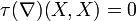$\tau(\nabla)(X,X) = 0$.

## Proof

The proof follows directly from the definition, and the fact that the Lie bracket of derivations is antisymmetric.